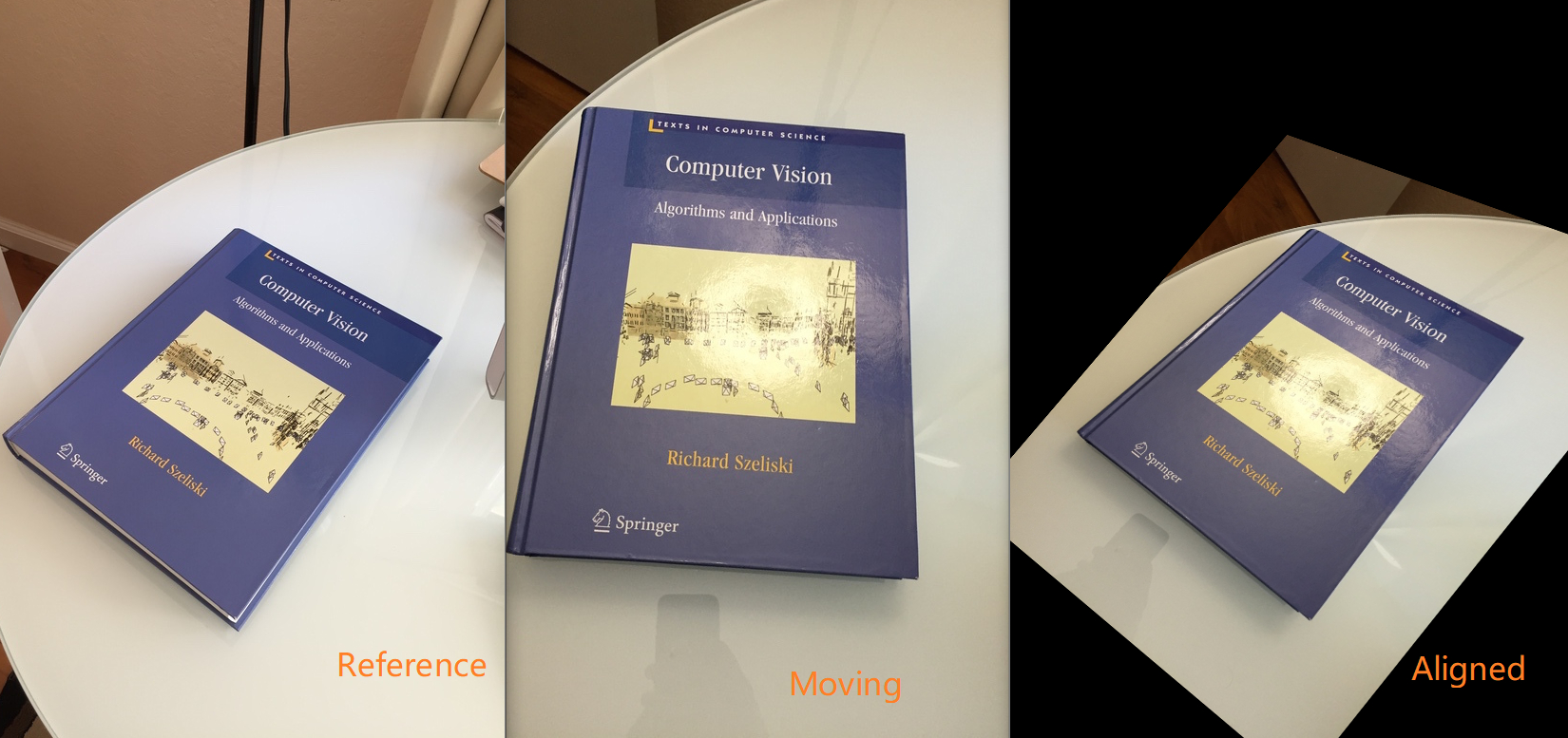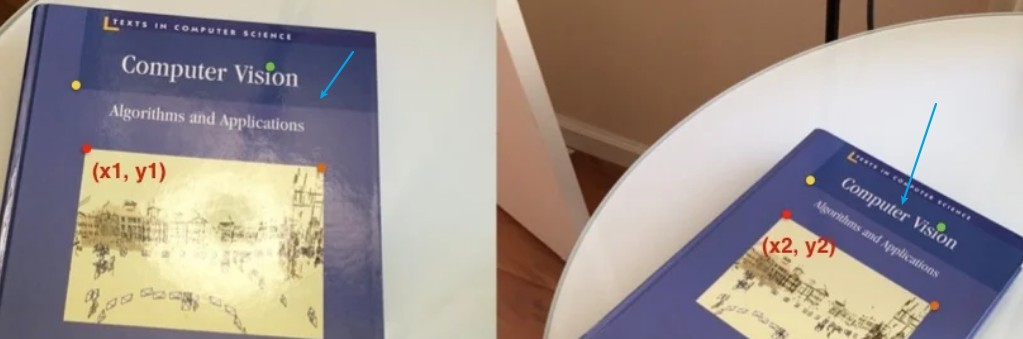# 不用PS 抠图，Python + OpenCV 自动实现海报场景替换！• 确定至少四组对应点坐标

• 找到一个转换矩阵；

• 把找到的转换矩阵应用到 Moving Image 上，实现图像对齐；$H = \left[ \begin{matrix} h_{00}& h_{01} & h_{02}\\ h_{10} & h_{11}&h_{12}\\ h_{20} & h_{21}&h_{22}\\ \end{matrix} \right]$
$A(x_1 ,y_1)$$A_1(x_2,y_2)$ 作为对应点，则 Homography $H$ 的的应用 如下：
$\left[ \begin{matrix} x_1\\ y_1\\ 1\\ \end{matrix} \right] = H\left[ \begin{matrix} x_2\\ y_2\\ 1\\ \end{matrix} \right] = \left[ \begin{matrix} h_{00} & h_{01} & h_{02}\\ h_{10} & h_{11} & h_{12}\\ h_{20} & h_{21} & h_{22}\\ \end{matrix} \right]\left[ \begin{matrix} x_2\\ y_2\\ 1\\ \end{matrix} \right]$

import cv2
import numpy as np

if __name__ =='__main__':

#图片读取
position_src = np.array([[141,131],[480,159],[493,630],[64,601]],dtype = float)

position_dst = np.array([[318,256],[543,372],[316,670],[73,473]],dtype = float)

#计算转换矩阵
h,status = cv2.findHomography(position_src,position_dst)

#对图片进行仿射变换
out_img = cv2.warpPerspective(img_src,h,(img_dst.shape,img_dst.shape))

#Display images;
cv2.imshow("Source image",img_src)
cv2.imshow("Destination Image",img_dst)
cv2.imshow("Warped Source Image",out_img)

cv2.waitKey(0)def mouse_handler(event,x,y,flags,data):
if event ==cv2.EVENT_LBUTTONDOWN:
cv2.circle(data['im'],(x,y),3,(0,0,255),5,16)
cv2.namedWindow("Image",0)
cv2.imshow("Image",data['im'])
if len(data['points']) <4:
data['points'].append([x,y])

def get_four_points(im):

data = {}
data['im'] = im.copy()
data['points'] = []
# Set the callback function for any mouse event
cv2.namedWindow("Image", 0)
cv2.imshow('Image',im)
#请注意你标记点的数据，是顺时针，需要与pst_src 方向一致
cv2.setMouseCallback("Image",mouse_handler,data)
cv2.waitKey(0)
# Convert array to np.array
#竖直方向堆叠起来;;;
points = np.vstack(data['points']).astype(float)
return pointsif __name__ =='__main__':

size = img_src.shape
# 取得四个坐标
pst_src = np.array(
[
[0,0],[size-1,0],
[size-1,size-1],
[0,size-1]
],dtype=float
)

print("Click on four corners of bllboard and the press ENTER")
four_point  = get_four_points(img_dst)

# Calculate  Homography between  source and destination points
h,status = cv2.findHomography(pst_src,four_point)

im_temp = cv2.warpPerspective(img_src,h,(img_dst.shape,img_dst.shape))

cv2.fillConvexPoly(img_dst,four_point.astype(int),0,16)

#add wraped source image to destination image

img_dst = img_dst + im_temp
cv2.namedWindow("Image", 0)
cv2.imshow("Image",img_dst)
cv2.waitKey(0)08-044万+

#### 基于OpenCV-python3实现抠图&替换背景图

07-162万+

#### opencv 截取轮廓中的图像——实现PS中的抠图功能 Opencv extract area circled by contour

07-244494

#### Python实现自动抠图（上篇）

02-207051

#### 使用opencv实现自定义抠图

04-2467

#### openCV实现类似ps抠图

08-104895

#### PS:换背景天空（简单抠图）

05-0248

#### PS怎么制作有透明通道的图片，保存为什么格式？

08-03

09-10138

#### PS 简单抠图流程

03-01539

#### ps抠图-基础篇（一）

12-211万+

#### opencv实现抠图（单一背景），替换背景图

11-06316

#### PS技术不够，Python来凑！python实现人工智能Ai抠图功能

07-19612

#### OpenCV - GrabCut 算法抠图（Python实现）

09-29323

#### 不会PS没关系，用python实现人工智能Ai抠图！

03-3093

#### opencv-python 使用掩模抠图

12-132616

#### ps-黑底图片抠图

06-272067

#### Photoshop抠图（用调整边缘命令抠图）

12-121486

#### ps-头发丝抠图

04-212496

#### Ps小技术——通道抠图大法

03-1831

#### PS快速秒抠图技巧©️2019 CSDN 皮肤主题: 大白 设计师: CSDN官方博客点击重新获取扫码支付